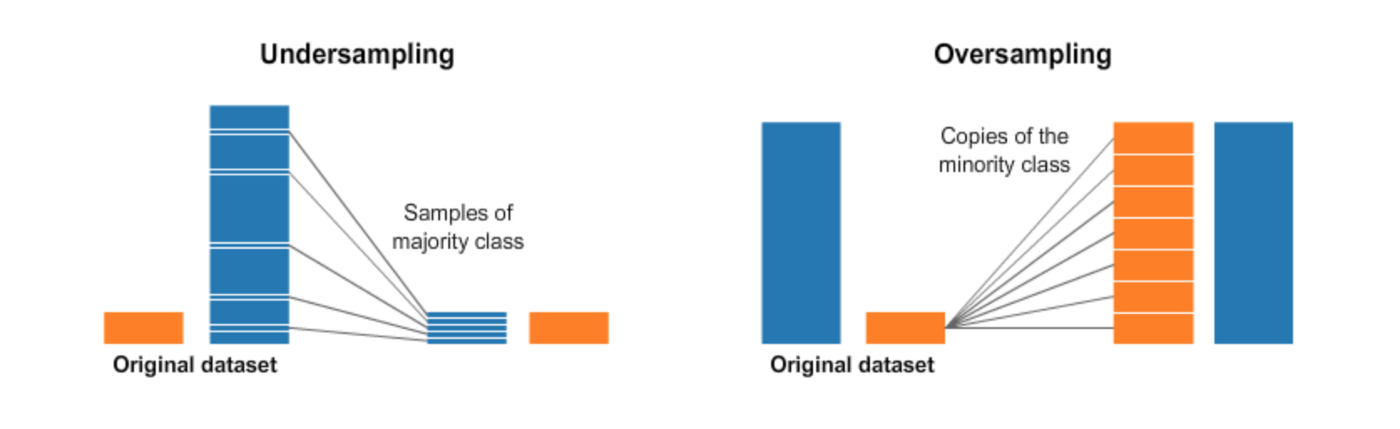Imbalanced datasets are a common problem in classification tasks in machine learning. Take credit card fraud prediction as a simple example: the target values are either fraud (1) or not fraud (0), but the number of fraud (1) could only be less than one percent of the whole dataset.

In this case, any model could "predict" all customers will not default and easily get 99% accuracy. This is due to most algorithms are designed to reduce error.

There are several commonly used approaches to deal with such problem. Namely two groups: resampling and ensembling.## 1. Undersampling / Downsampling

Undersampling is the process where you randomly delete some of the observations from the majority class in order to match the numbers with the minority class.

Undersampling would be helpful if the minority class contains decent amount of data. Otherwise, undersampling would make the dataset quit small. Further, the data we are dropping could be important.

## 2. Oversampling / Upsampling

Oversampling is the process that reproduce data for the minority class to match the number of observations in the majority class. Oversampling can be a good choice when the minority class contains few observations.

Important: Always split into test and train sets BEFORE trying oversampling techniques! Oversampling before splitting the data can allow the exact same observations to be present in both the test and train sets. This can cause overfitting and poor generalization to the test data.

There are several ways to generate data for the minority class. Following is a simple example to oversample the minority class:

from sklearn.utils import resample

# define features and target
X = df.drop('Target', axis=1)
y = df['Target']

# split data into training and test sets (80% - 20%)
X_train, X_test, y_train, y_test = train_test_split(X, y, test_size=0.2, random_state=42)

# concatenate training data
X = pd.concat([X_train, y_train], axis=1)

# define minority and majority classes
not_fraud = X[X.Class==0]
fraud = X[X.Class==1]

# upsample minority
fraud_upsampled = resample(fraud,
replace=True, # sample with replacement
n_samples=len(not_fraud), # match number in majority class
random_state=27) # reproducible results

# combine majority and upsampled minority
df_train_up = pd.concat([not_fraud, fraud_upsampled])

# check new class counts
df_train_up.Target.value_counts()
1    176534
0    176534

# reassign training data
y_train = df_train_up['Target']
X_train = df_train_up.drop('Target', axis=1)


### SMOTE

There is a widely used oversampling technique called SMOTE (Synthetic Minority Over-sampling Technique). In simple terms, it looks at the feature space for the minority class data points and considers its k-nearest neighbours.

Again, it’s important to generate the new samples only in the training set to ensure our model generalizes well to unseen data.

from imblearn.over_sampling import SMOTE

# Separate input features and target
y = df.Class
X = df.drop('Class', axis=1)

# setting up testing and training sets
X_train, X_test, y_train, y_test = train_test_split(X, y, test_size=0.25, random_state=27)

sm = SMOTE(random_state=27, ratio=1.0)
X_train, y_train = sm.fit_sample(X_train, y_train)


## 3. sklearn's Approach

The Scikit-Learn package provides a integrated way to tackle this problem by setting up the class_weight='balance'. This is supported by several classifiers like decision tree.

clf_lr = LogisticRegression(random_state = 42,
solver='liblinear',
class_weight='balanced')
clf_lr.fit(X_train,y_train)